Journal DetailsFormat
Journal
eISSN
2083-134X
First Published
16 Apr 2011
Publication timeframe
4 times per year
Languages
EnglishOpen Access

# The impact of medium frequency pulsed magnetron discharge power on the single probe Langmuir measurements and resulted plasma parameters

###### Accepted: 22 Nov 2015
Journal DetailsFormat
Journal
eISSN
2083-134X
First Published
16 Apr 2011
Publication timeframe
4 times per year
Languages
English

The resonant type power supplies of medium frequency designed for magnetron sputtering processes often use pulse density modulation to regulate the average discharge power level. While the output power level changes then number of pulses in a group changes, but the discharge current pulses are the same from pulse to pulse: their parameters (duration time, amplitude) do not change with the discharge power. The goal of this paper is to present the influence of medium frequency discharge power level on the direct current I-V characteristics of a single Langmuir probe and resulting plasma parameters caused by the pulse density modulation. The sputtering processes of titanium and copper were diagnosed at two spatial positions. The measured Langmuir probe I-V characteristics showed strong dependence on the discharge power. As the discharge powering pulses stay the same with the discharge power level change, such influence was unlikely to occur. Using time-resolved analysis of probe current waveforms the origin of this influence was indicated. The influence of discharge power level on the single probe Langmuir I-V characteristics and resulting plasma parameters was eliminated using a simple method of scaling the results. Finally, the reliable plasma parameters were calculated.

#### Keywords

Introduction

Magnetron sputtering techniques are widely applied in industrial deposition processes of thin films and advanced materials development or treatment [1-4]. Through the years of research and scientific exploration a great variety of magnetron designs and their powering have been developed with a great interest in pulsed magnetron sputtering at present time [5-13]. The one thing all magnetrons have in common is that energetic electrons are confined near the target by a combination of electric and magnetic fields. This combination forces the energetic electrons to execute a complex movement, including mainly cyclotron motion (gyration) and E × B closed-drift motion (Hall current). The electrons with lower energy (typically a few eV) are not able to penetrate the cathode sheath but “bounce off” and return to the plasma . In many applications it is necessary to know the parameters of magnetron plasma (plasma of magnetically enhanced glow discharge) to ensure reproducibility of technological processes . Various diagnostic tools may be used to achieve this aim, including electrostatic probes collecting charged particles from discharge plasma [15-18].

The simplicity of a single Langmuir probe measurement equipment, consisting of regulated voltage source, ammeter and conductive tip immersed in plasma, makes this technique very attractive. In principle, the current-voltage (I-V) characteristic of a single Langmuir probe can provide (after some data processing) electron density ne, electron temperature Te and plasma potential Vp [19, 20]. Unfortunately, the measured I-V data are often affected by the experimental conditions and interpretation of Langmuir probe I-V characteristic is not straightforward in many cases [22, 23, 25]. First of all the effects of magnetic field presence [21, 24, 26, 44], geometrical characteristics of the probes [27, 28] and discharge power [29, 30] have to be taken into account to calculate reliable plasma parameters from Langmuir probe I-V characteristic. Fortunately, considering experimental conditions of magnetron sputtering deposition processes, the plasma parameters may be calculated from Langmuir probe I-V characteristic basing on quite simple equations and procedures, even regarding time-resolved analysis [18, 20, 28, 30-33, 41-43].

In this paper we present the impact of medium frequency pulsed magnetron discharge power on the I-V characteristics of single Langmuir probe and resulting plasma parameters caused by the pulse density modulation (section 2.1). The pulsed powering of magnetron introduces discharge ‘ON’ and ‘OFF’ phases, which results in plasma presence and absence phases, respectively. One can conclude that this fact has a great impact on the measured Langmuir probe current if its time-averaged value (i.e. its mean value, direct current) is recorded . It may be stated that, regarding pulsed powering of magnetron source, the magnetron current waveform has to be considered as another factor that influences the direct current I-V characteristics of Langmuir probe.

Experimental

The sputtering processes discussed in this paper were performed in a vacuum system equipped with a rotary and diffusion pump with a pumping speed of 2000 l/s. The final pressure in the deposition chamber was about 1 × 10–5 hPa. The working pressure of argon during the experimental magnetron sputtering processes was about 4 × 10–3 hPa.

The circular planar magnetron source WMK-50 (designed and developed at the Wroclaw University of Technology, Poland) with a target of W1/2 = 25 mm in radius was used during the experiments. Because of extremely efficient cooling system the WMK-50 magnetron source is capable of operating at a target power density of up to 1000 W/cm2. That was one of the essentials to reach the previously reported [34, 35] pulsed self-sustained self-sputtering mode of magnetron operation. In the present work, the results obtained during two sets of sputtering processes are presented: first using titanium and second using copper target. Both targets were 7 mm thick and both had erosion zone depth (racetrack depth) of about 2.5 mm. The magnetic field induction value (component parallel to the target surface) was about 120 mT at the bottom of erosion zone. The magnetic null point of the WMK-50 magnetron source was measured to be placed at the distance of ZBZ = 39 mm from the target surface above the center magnetic pole piece. Using the Gencoa Ltd. classification of balanced/unbalanced magnetrons with parameter g = ZBZ/W1/2 the magnetron source used during the experiments is the group III device (g = 1.56) i.e. medium balanced .

The sputtering equipment

The magnetron sputtering source was powered by a medium frequency (MF) power supply: Dora Power Systems MSS-14 . The MSS-14 is the resonant type power supply. The output current pulses are sinusoidal-shaped with the frequency set at about 120 kHz, which corresponds to a single MF pulse duration of about 8.3 µs (Fig. 1). The design of the power supply ensures its stable operation in a wide load impedance range, from open to short circuit at the output. At high load impedance (ignition phase of magnetron discharge) the power supply operates as a voltage source with a maximum output voltage of about 1200 V, while at low load impedance (magnetron discharge after ignition) it operates as a current source with sinusoidal-shaped output current pulses of about 14 A rms (amplitude of about 19 A). The amplitude of the output current pulses is stabilized thanks to the electronic circuit that maintains a constant value of the Q-factor of the series resonant power circuit [37, 38]. For this reason, the amplitude of the output current pulses is a fixed value that correlates with the L and C reactance elements of the above mentioned series resonant power circuit and is independent of load impedance (of course up to maximum output voltage only, i.e. 1200 V). The shape of the load voltage pulses results from the sinusoidal-shaped current waveform and load impedance as long as this voltage is lower than the maximum output voltage. If technological parameters of sputtering process are fixed (pressure, cathode material, magnetic induction value) then the magnetron impedance variation is the same for each pulse. As a result, the shape of discharge voltage pulses is invariable from pulse to pulse (Fig. 1).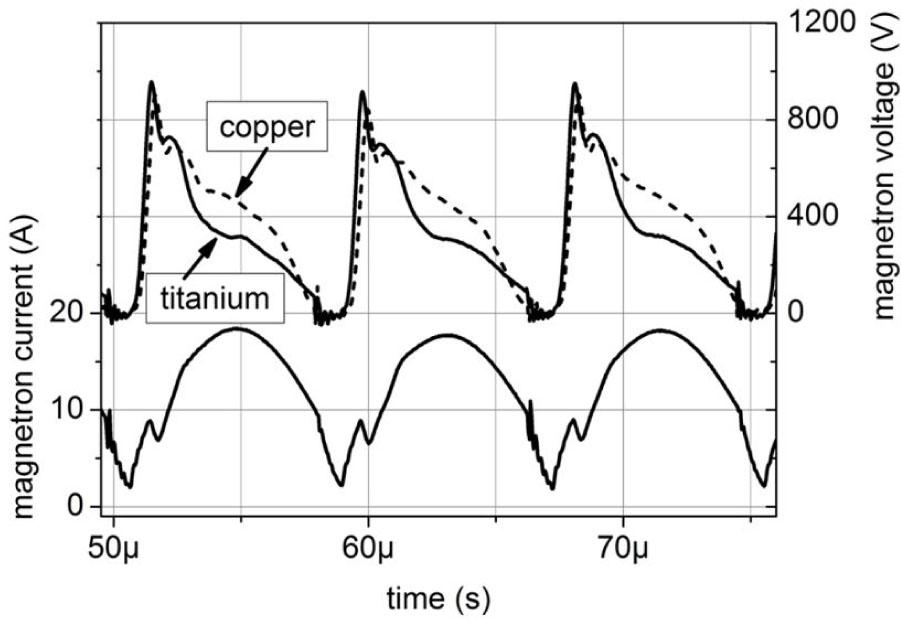The WMK-50 magnetron source current and voltage waveforms while driven by the MSS-14 power supply. The voltage waveforms show the difference in the discharge voltage pulses caused by different values of the target material ISEE (Ion induced Secondary Electron Emission) coefficient.

To regulate the average output power level (discharge power level) the pulse density modulation (PDM) is used (Fig. 2). Output current pulses are gated with frequency of about 550 Hz, its inverse gives the group repetition time of about Tgating = 1.8 ms. The increase of power delivered to the magnetron source is accomplished by the increase of pulse number in each group. As it was mentioned above, the parameters of output current pulses stay the same, they do not change with the output power level change. The increase of the output power increases linearly the group duration time (tgroup) only. For an example titanium sputtering processes at discharge power of 1, 3 and 5 kW the mean group duration time was of about 0.24, 0.72 and 1.20 ms, respectively (Fig. 2). The mean group duration time was calculated over 128 groups of pulses using Agilent DSO-X 3014A oscilloscope. In case of copper sputtering, the mean group duration time (at the same power levels as above) was of about 0.2, 0.6 and 1.0 ms, respectively. The difference in group duration time for those materials results from the discharge voltage difference caused by different values of argon ion induced secondary electron emission coefficient, ISEE(Ti) = 0.11, ISEE(Cu) = 0.08 electron per ion, for argon ion energy of about 500 eV . With the ISEE(Cu) being lower than ISEE(Ti), the discharge voltage is higher in case of copper sputtering (Fig. 1). Taking into account invariant discharge current pulses (constant amplitude, duration time) one can conclude that higher energy is delivered to the magnetron source with each pulse while sputtering copper than titanium. To keep the same average discharge power, the number of pulses in a group has to be lower in case of copper sputtering. The group duration time may slightly vary from its mean value (easy to be seen in Fig. 2, waveforms 1 kW and 5 kW) as the technological parameters (e.g. pressure) dynamically change during the magnetron sputtering process [7, 45].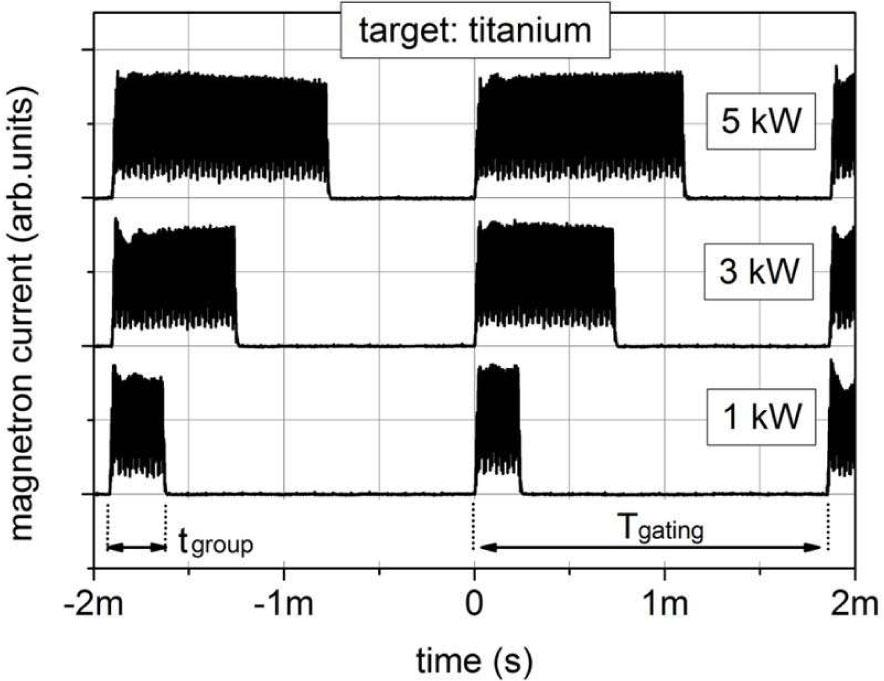Waveforms of the MSS-14 power supply output current pulses while driving the WMK-50 magnetron equipped with titanium target. The time point of zero indicates the trigger moment of the oscilloscope time base.

One can see that the output current pulses of MSS-14 power supply do not reach the zero value (Fig. 1, Fig. 2). This is because the series resonant power stage is driven by the MOSFET H-bridge with the signal of frequency little lower than its resonant frequency given by the L and C values. That makes the resonant circuit impedance having capacitive component. As a result of that, the inductive component of the power supply load impedance (glow discharge of magnetron source) that influences the resonant circuit may be compensated even at high output power level . Since the MSS-14 operates as a current source, its maximum output power (at the group duration time equal to the group repetition time) depends on the load impedance. In case of titanium and copper sputtering processes discussed in this paper, the maximum discharge power was of about PE_max ≈ 7.5 kW and 9 kW, respectively. The output power limit of the MSS-14 power supply, resulting from its design, is of about 14 kW.

The Langmuir probe

The plasma diagnostic was performed using a single cylindrical Langmuir probe with a tungsten tip of Rprobe = 0.2 mm in radius and active length, exposed to the plasma, of 3 mm. The 20 mm long tip holder was designed similarly to the one presented in [33, 43, 44]. The two co-axial pipes (inner made of ceramics, outer made of stainless steel) were used to form re-entrant structure in such a way that the sputtered material could not short circuit the probe tip to the holder surface. The outer radius of the tip holder was of Rholder = 1.25 mm. The probe tip was oriented perpendicular to the target surface to minimize its coverage with sputtered atoms [40-42]. The target to probe distance was 60 mm. As the distance of the probe to cathode voltage drop region of the discharge is large it may be assumed that the changes of the space potential along the length of the probe are insignificant and may be neglected . The previous experiments with a Langmuir probe in copper sputtering deposition processes, in which the MSS-5 (maximum discharge power of about 5 kW) power supply and the WMK-50 magnetron source were used, showed the maximum electron temperature and plasma density of about 1.3 eV and 4 × 1016 m–3, respectively . Taking into account those results and actual usage of MSS-14 power supply (higher discharge current and power), the electron temperature and plasma density ranges of variability were assumed to be 0.5 to 5 eV and 1016 to 1017 m–3 respectively. For such plasma parameters the Debye screening length is of about λD = 0.02 to 0.2 mm. Regarding the assumed plasma parameters and probe dimensions, both the Debye shielding length and the probe radius meet the condition of being lower than electron-neutral and ion-neutral mean-free-paths, that are expected to be not less than ∼10 mm [33, 43]. It may be stated that the experimental setup fulfils the basic requirements for Langmuir-probe diagnostics given in . The only inconvenient thing is that the Debye ratio ξ = RprobeD is in the range of about 10 > ξ > 1 and a careful selection of ions collection theory is needed if precise values of plasma parameters are to be calculated (if precise values are possible to be calculated at all) . Since this paper focuses on the impact of power supply features on the measured Langmuir probe I-V characteristics, a simple ion saturation current evaluation was only performed (Section 3), in spite of the fact that systematic error was introduced because of that. The Langmuir probe measurements were performed at two spatial positions with respect to the center point of the target (Fig. 3). Position No. 1 is directly over the center magnetic pole piece, position No. 2 is 60 mm away from the first position in a radial distance. The magnetic field induction B (components parallel and perpendicular to the target surface) at both probe positions were lower than 0.6 mT. The plasma ions were assumed to be unaffected by the magnetic field .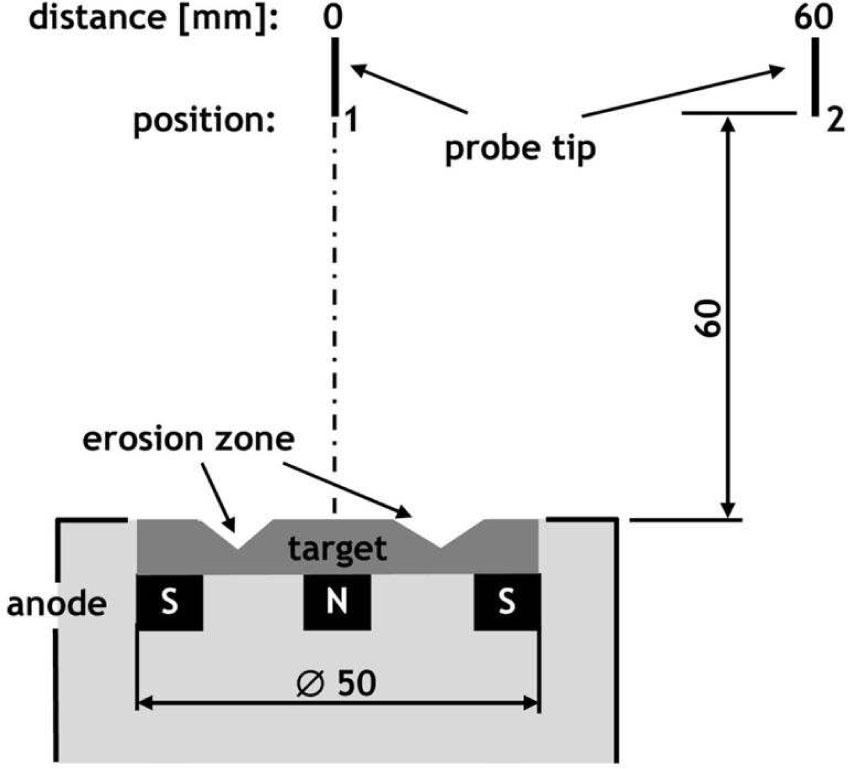Details of the Langmuir probe measurements arrangement indicating two chosen spatial positions.

The plasma electrons may be assumed to be non-magnetized, since the ratio of the probe radius Rprobe to the mean Larmor radius for electrons having Maxwellian energy distribution function, rLe = (πmekTe/2)1/2/eB [24, 33] is Rprobe/rLe < 0.1 for electron temperature down to Te ≈ 0.2 eV, where e denotes elementary charge, k is the Boltzmann constant, me is the electron mass.

The average values of Langmuir probe current were measured using DC ammeter incorporated into Agilent U1272A multimeter. With respect to the pulsed magnetron sputtering process, where the peak values of the probe current are much higher than its average value it may be problematic to do the correct measurement of this average value using a simple digital ammeter. Prior the experiments, the U1272A meter was successfully tested in terms of its capability of proper measuring of such signal. The readings of U1272A DC ammeter were compared with the time-averaged value of current calculated by the oscilloscope for a wide range of values: from single µA to tens of mA. The time-resolved Langmuir probe current waveforms were recorded using Agilent DSOX 3014A (100 MHz) oscilloscope equipped with N2774A (DC to 50 MHz) current probe. The oscilloscope time base triggering was synchronized with the signal gating the MSS-14 output current pulses.

The Langmuir probe measurements

With respect to pulsed magnetron sputtering deposition processes, the time-resolved evolution of plasma parameters (ne, Te, Vp) = f(t) during an individual discharge pulse give the broad information regarding plasma physics and energetic conditions of film growth [41-43]. Despite this, the time-averaged values of those parameters may also provide useful information e.g. for comparison purposes . Furthermore, the apparatus for time-resolved Langmuir probe measurements is usually quite expensive in comparison to Langmuir probe systems that measure time-averaged values. In case of power supply that regulates output power level using PDM modulation (like Dora Power System MSS-14) the time-averaged Langmuir probe current, measured at given probe voltage, is influenced by the ratio of group duration time with respect to group repetition time (this issue will be discussed closely in Section 4). Basically, the reason for this is the fact that averaging over the time includes groups of pulses (discharge presence periods) and no discharge presence periods that take place between these groups (Fig. 2). Because of that the measured Langmuir probe I-V characteristics (and resulted, calculated plasma parameters) are expected to show strong dependence on the discharge power level. For a given target material and fixed other technological parameters, this dependence is confusing since it suggests the plasma energetic conditions being a subject of changes with the discharge power level. As described in Section 1 the shapes of the discharge current and voltage pulses are unaffected by the discharge power, so the plasma parameters should be unaffected by the discharge power too (ignoring the effects of background gas heating ). In such case the reliable time-averaged plasma parameters may only be calculated from Langmuir probe I-V characteristics measured at a condition of group duration time being equal to the group repetition time tgroup = Tgating, i.e. at the condition of maximum discharge power. At such condition the magnetron current waveform is a continuous train of pulses and averaging probe current over the time includes discharge presence periods only. The Langmuir probe current value (at a given probe voltage) represents then its average value measured over the discharge pulses only. Unfortunately, this is the case of maximum discharge power range of about 10 kW in our experiments, as given in Section 2.1. High discharge power makes Langmuir probe measurements difficult or even impossible to be done. The main problem arises from the fact of excessive probe tip heating during diagnosis of electron saturation region of the probe I-V characteristics . While the tip temperature increases, the probe may emit electrons (perturbations are introduced to the plasma being measured) or may become melted (destruction of measuring tool). One may conclude that ion saturation region of the probe I-V characteristics should be diagnosed instead of electron saturation region, but in the case of ion saturation region another problems arise. First of all, if the ion density (plasma density assuming plasma quasi-neutrality) is to be calculated then the ion mass has to be known. As the average or pulse discharge power is high then a significant contribution of the self-sputtering phenomenon to the discharge maintaining mechanisms should be taken into account [7, 29, 35]. It was published previously  that contribution of the self-sputtering phenomenon to the discharge maintaining mechanisms may be sufficiently high to sustain the pulsed magnetron discharge after argon flow had been cut off. The continuous process of pulsed self-sustained self-sputtering of copper was successfully obtained using WMK-50 magnetron source powered by the MSS-14 power supply (the same devices that have been used in the present work). The continuous self-sustained self-sputtering mode of magnetron operation means pure metallic plasma (the only plasma ions are the sputtered cathode material ions). Taking into account that the ionization probability of sputtered cathode atoms may be significant, at high discharge power, and the plasma being diagnosed is composed not only of process gas ions (argon) a question is to be answered: what is the plasma ion mass? A clear answer is difficult to be given, so the plasma density calculated using ion saturation region of probe I-V characteristic suffer on that. Furthermore, high discharge power (high peak values of magnetron current) makes it difficult to diagnose the ion saturation region as the influences of parasitic electromagnetic interferences and feedbacks often decrease the probe current measurement accuracy [30, 41].

The issues mentioned above, that refer to our experimental conditions, point out that one reasonable way to obtain the reliable time-averaged plasma parameters is to do the Langmuir probe measurements at moderate discharge power PE, then calculate the electron current curve Ie(Vprobe, PE) and scale it up to the maximum discharge power PE_max (group duration time equal to the group repetition time) which let us determine the not measurable curve of electron current Ie(Vprobe). Finally, we can calculate the plasma parameters using the obtained electron current curve. Another way to obtain the reliable time-averaged plasma parameters is to do the time-resolved measurements at moderate discharge power PE, then calculate the time-averaged plasma parameters by averaging over single discharge pulses. This approach employs the usage of an expensive apparatus for time-resolved Langmuir probe measurements whereas our aim was to carry out such measurements using inexpensive Langmuir probe system that measures time-averaged values.

The Langmuir probe I-V characteristics were measured at discharge power of 1, 3 and 5 kW for both titanium and copper sputtering processes. The ion and electron saturation regions were diagnosed using probe voltage Vprobe up to – 150 V and +100 V, respectively (with respect to the grounded body of the vacuum vessel). The positive probe voltage was reduced, in some cases, as it was needed to prevent the probe tip from excessive heating (especially at probe position No. 1). To obtain the electron current value one has to subtract the ion saturation current from the measured probe I-V characteristics . Because the Debye ratio was expected to be 10 > ξ > 1 in our experiments and ion mass was difficult to be clearly defined the ion saturation current was estimated using a simple approach presented in . It is based on the assumption that if electron energy distribution function (EEDF) is Maxwellian then the correct ion saturation current value Iis is subtracted from measured probe I-V characteristic if the linear part of resulting curve (retardation of electrons) on a semi-logarithmic plot extends over as large voltage range as possible. A systematic error may be introduced because of that simple approach. This error may affect absolute values of calculated plasma parameters, but it should be emphasized that such approach for ion saturation current determination does not affect the principles of the analysis presented further.

Scaling of Langmuir probe IV characteristics

The electron current curves Ie(Vprobe, PE) calculated from the measured probe I-V characteristics are shown in Fig. 4 (probe position No. 1) and Fig. 5 (probe position No. 2). One can easily see the strong dependence of electron current on the discharge power, PE. This may suggest that the plasma parameters are subjects to change with the discharge power change. As it was stated earlier such effect is unlikely to take place, because the discharge powering pulses do not change with the discharge power. The explanation of this observed effect is that the measured probe I-V characteristics were influenced by the magnetron powering waveform, since the time averaged values of probe current were recorded. As a result, the electron current curves depicted in Fig. 4 and Fig. 5 differ with the discharge power level, following the relation:

$Ie(Vprobe,PE)∼Ie(Vprobe)tgroup(PE)Tgating ∼Ie(Vprobe)PEPE_max$$$\matrix{ {{I_e}\left( {{V_{probe}},{P_E}} \right)\sim {I_e}\left( {{V_{probe}}} \right){{{t_{group}}\left( {{P_E}} \right)} \over {{T_{gating}}}}} \cr {\,\,\,\,\,\,\,\,\,\,\,\,\,\,\,\,\,\,\sim {I_e}\left( {{V_{probe}}} \right){{ {{P_E}} } \over {{P_{E\_}}_{max}}}} \cr }$$

As long as the given cathode material and probe position are considered, then with equation 1 it may be concluded (and it is reflected in Fig. 4 and Fig. 5) that the discharge power increase causes the electron current curves to be shifted along the current axis. Unfortunately, the equation 1 is not complete, since the probe current value (at a given probe voltage) does not change exactly with the same ratio as the target power changes. It is depicted in Fig. 6 for the electron current saturation regions of the curves from Fig. 4 and Fig. 5.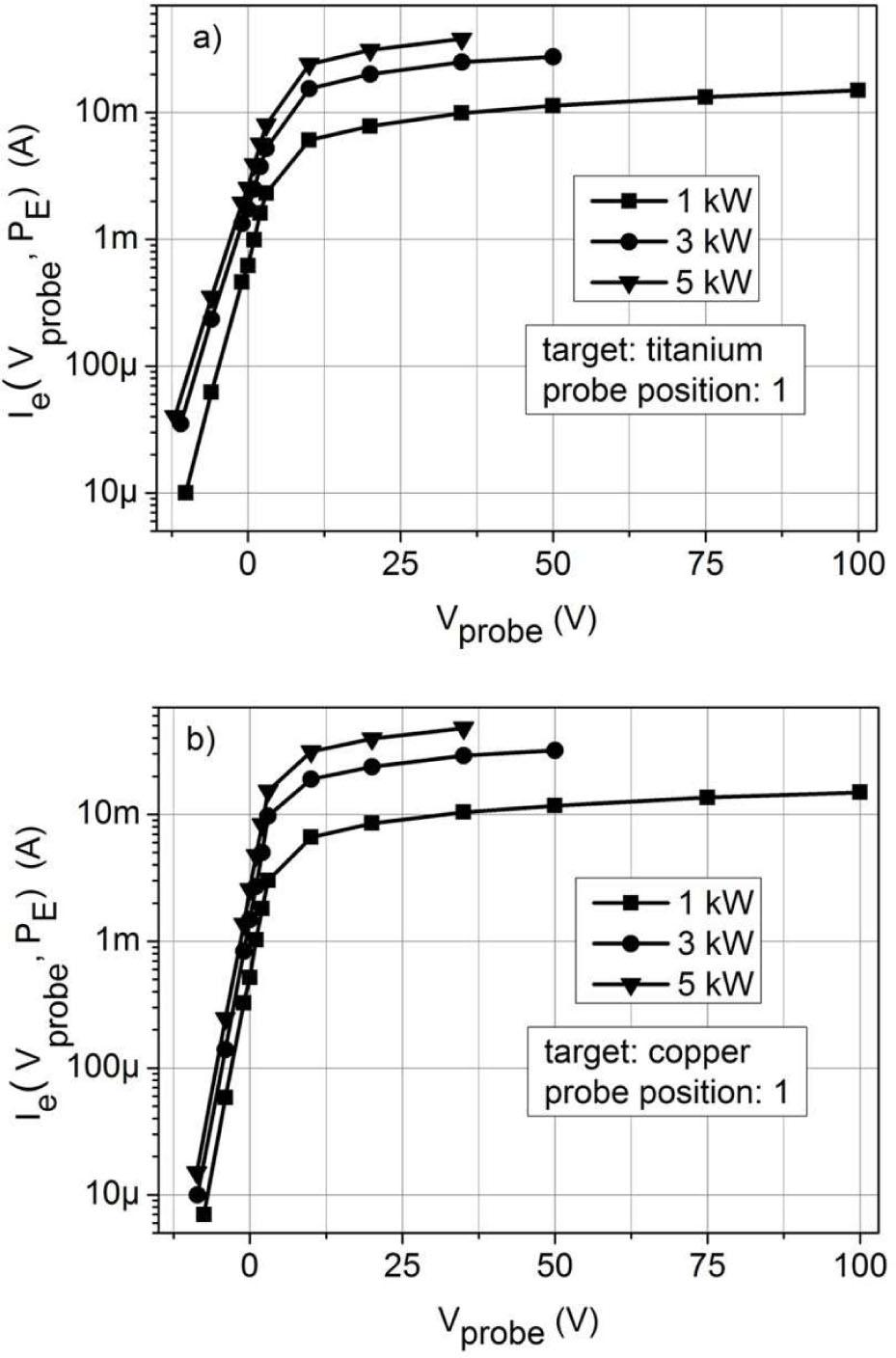The electron current curves calculated from Langmuir probe I-V characteristics measured at position No. 1 for (a) titanium and (b) copper sputtering processes, at discharge power of 1; 3; 5 kW.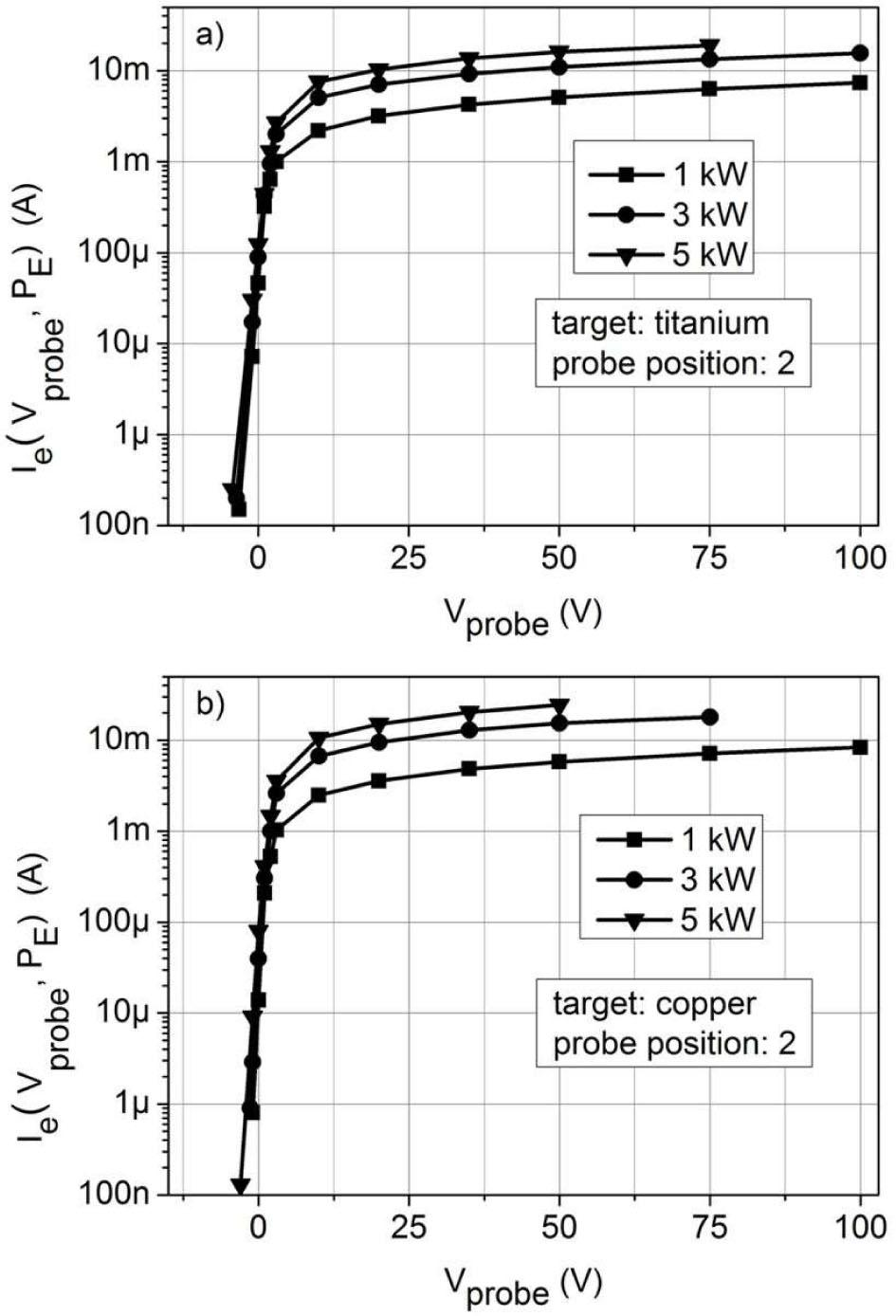The electron current curves calculated from Langmuir probe I-V characteristics measured at position No. 2 for (a) titanium and (b) copper sputtering processes, at discharge power of 1; 3; 5 kW.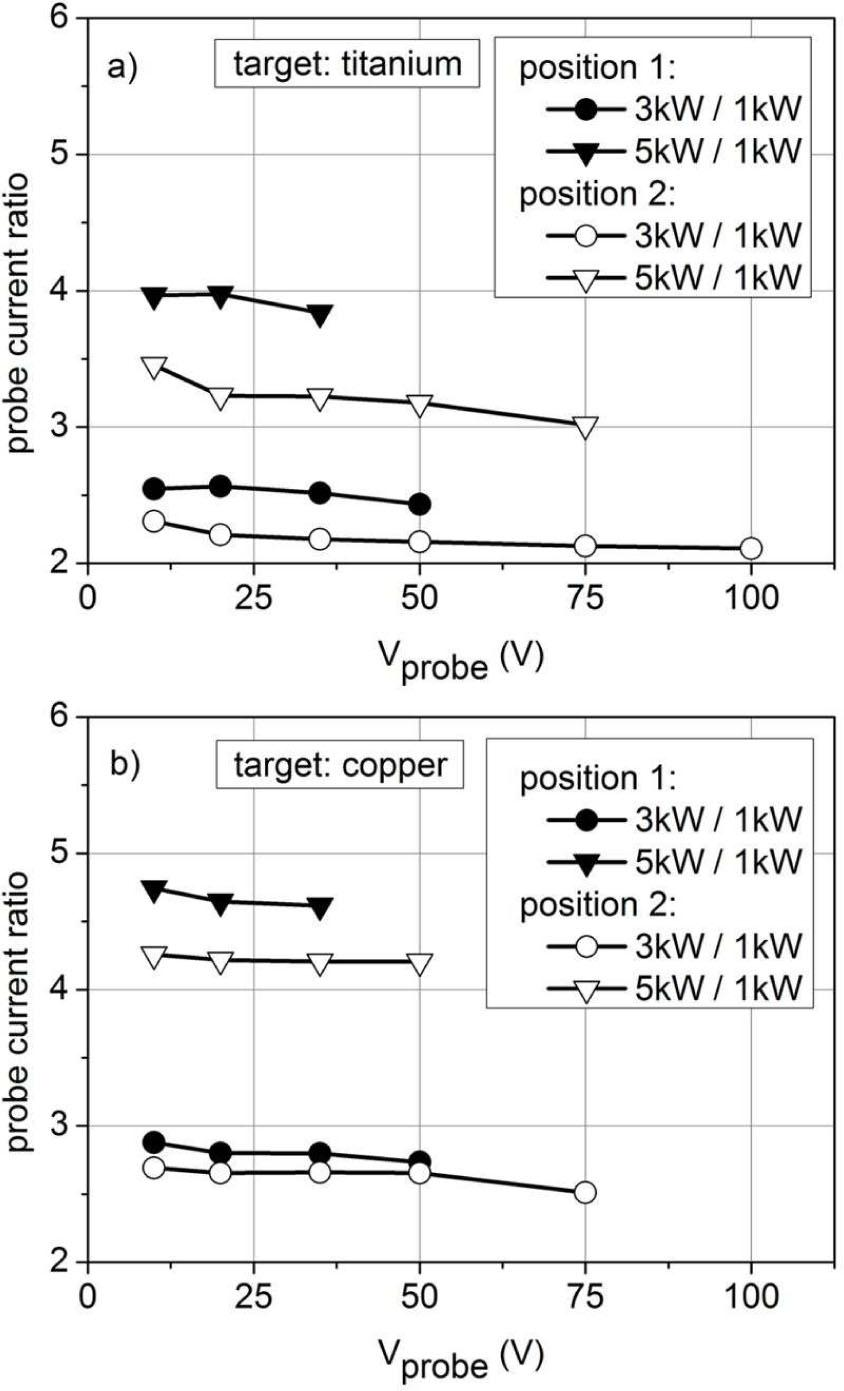The electron current ratios calculated in electron saturation regions of probe I-V characteristics for (a) titanium and (b) copper sputtering processes.

The expected values of probe current ratio, predicted by equation 1, with the discharge power change from 1 to 3 kW and from 1 to 5 kW were 3 and 5, respectively. In case of the copper sputtering processes the values of 2.5 to 2.9 and 4.2 to 4.7 were obtained instead of 3 and 5, respectively. In case of the titanium sputtering processes it was even worse, as the values of 2.1 to 2.6 and 3 to 4 were obtained instead of 3 and 5, respectively. This suggests that equation 1 does not correctly describe the influence of magnetron powering waveform on the measured Langmuir probe current (average values). To have a closer look, the time-resolved measurements of the Langmuir probe current Ip(t) were performed (Fig. 7). The Langmuir probe current oscillograms were recorded simultaneously with the magnetron current oscillograms. In general, the recorded Langmuir probe current waveforms showed similar relation with the target power change as the magnetron current waveforms depicted in Fig. 2, but some details influencing the mean probe current values were revealed.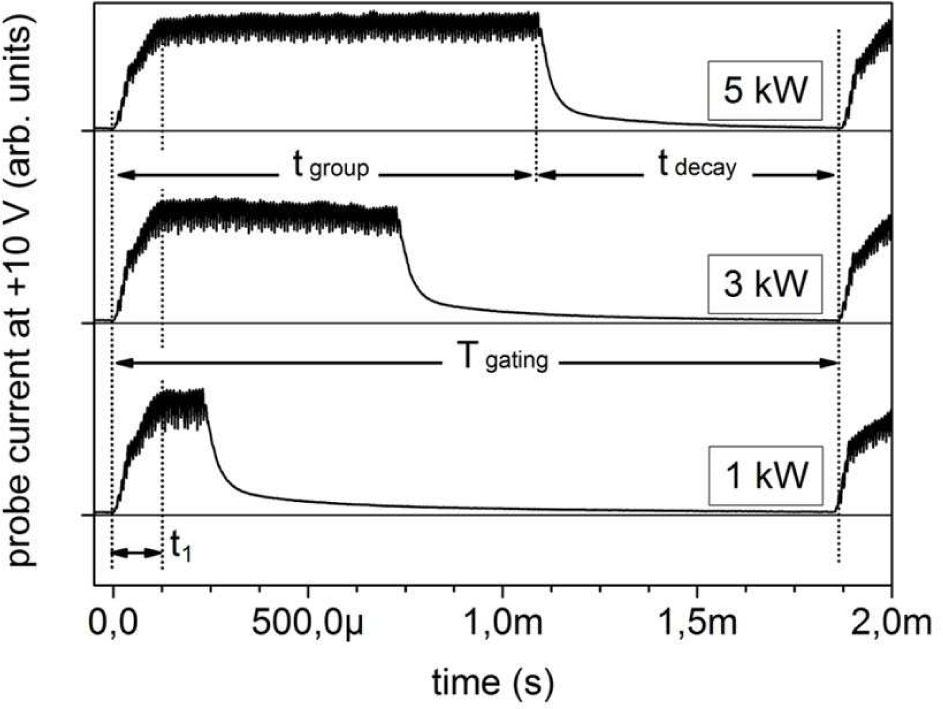Langmuir probe current waveforms recorded during titanium sputtering at +10 V of probe voltage.

As one can see in Fig. 7 there are two stages of magnetron discharge that can be distinguished during the group duration time tgroup. The first one is the discharge start-up phase. The duration of this phase is independent of the discharge power level and in case of the experiments discussed here it takes about t1 ≈ 125 µs. That corresponds to target power level of about 500 W. The second phase is the “steady state” of pulsed sputtering of the cathode, and it lasts for tgroup – t1. The probe current changes observed here indicate the changes of plasma parameters according to the actual value of the discharge current. As the discharge powering pulses discontinue at tgroup time, then the discharge plasma decay starts. Assuming an exponential decay, its time constant τdecay is of about 80 μs. Taking the mentioned observations into account, the mean electron current value (at given probe voltage Vprobe and discharge power PE) is defined as

$Ie(Vprobe,PE)=1Tgating[∫0t1Ip(t)dt+ ∫t1tgroupIp(t)dt+∫tgrouptgatingIp(t)dt+]−Iis$\eqalign{ & {I_e}\left( {{V_{probe}},{P_E}} \right) = {1 \over {{T_{gating}}}}\left[ {\mathop \smallint \limits_0^{{t_1}} {I_p}\left( t \right)dt + \,\,\mathop \smallint \limits_{{t_1}}^{{t_{group}}} {I_p}\left( t \right)dt} \right. \cr & \left. { + \mathop \smallint \limits_{{t_{group}}}^{{t_{gating}}} {I_p}\left( t \right)dt + } \right] - {I_{is}} \cr}

If the group duration time is tgroup > 125 µs (the target power level is higher than 500 W) then the first integral will have a constant value, independent of the target power level. If the time tdecay = Tgating – tgroup, left between the successive groups of pulses for the plasma to decay, satisfies the condition of tdecay ≫ τdecay then the third integral will also have a constant value, independent of the target power level (with a good approximation). Subtracting the equation 2 written for two discharge power values PE2 >PE1 the constant values cancel, and the result is the mean value of probe electron current for the time period of tgroup(PE2) – tgroup(PE1), which comprises only the “steady state” of pulsed discharge:

$Ie(Vprobe,PE2)−Ie(Vprobe,PE1)=1Tgating∫tgroup(PE1)tgroup(PE2)Ip(t)dt$\eqalign{ & {I_e}\left( {{V_{probe}},{P_E}_2} \right) - {I_e}\left( {{V_{probe}},{P_E}_1} \right) = \cr & {1 \over {{T_{gating}}}}\mathop \smallint \limits_{{t_{_{group\left( {{P_{E1}}} \right)}}}}^{{t_{group\left( {{P_{E2}}} \right)}}} {I_p}\left( t \right)dt \cr}

Scaling this result up to continuous train of powering pulses one can obtain equation 4. As the discharge power is a linear function of the group duration time (Fig. 2), it is convenient to introduce the notation of equation 4 by usage of discharge power values (5):

$Ie(Vprobe)=fracTgatingtgroup(PE2)−tgroup(PE1)×[Ie(Vprobe,PE2)−Ie(Vprobe,PE1)]$$$\matrix{ {{I_e}\left( {{V_{probe}}} \right) = frac{T_{gating}}{t_{group}}\left( {{P_{E2}}} \right) - {t_{group}}\left( {{P_{E1}}} \right)} \hfill \cr { \times \left[ {{I_e}\left( {{V_{probe}},{P_{E2}}} \right) - {I_e}\left( {{V_{probe}},{P_{E1}}} \right)} \right]} \hfill \cr }$$$Ie(Vprobe)=PE_maxPE2−PE1×[Ie(Vprobe,PE2)−Ie(Vprobe,PE1)]$$$\begin{gathered} {I_e}({V_{probe}}) = \frac{{{P_{E\_max}}}}{{{P_{E2}} - {P_{E1}}}} \hfill \\ \times \left[ {{I_e}\left( {{V_{probe}},{P_{E2}}} \right) - {I_e}\left( {{V_{probe}},{P_{E1}}} \right)} \right] \hfill \\ \end{gathered}$$

The equation 4 and equation 5 give quite consistent results as the obtained Ie(Vprobe) curves, calculated with different value pairs of the discharge power (PE1:PE2) = (1:3); (1:5); (3:5) kW, are difficult to be distinguished (Fig. 8).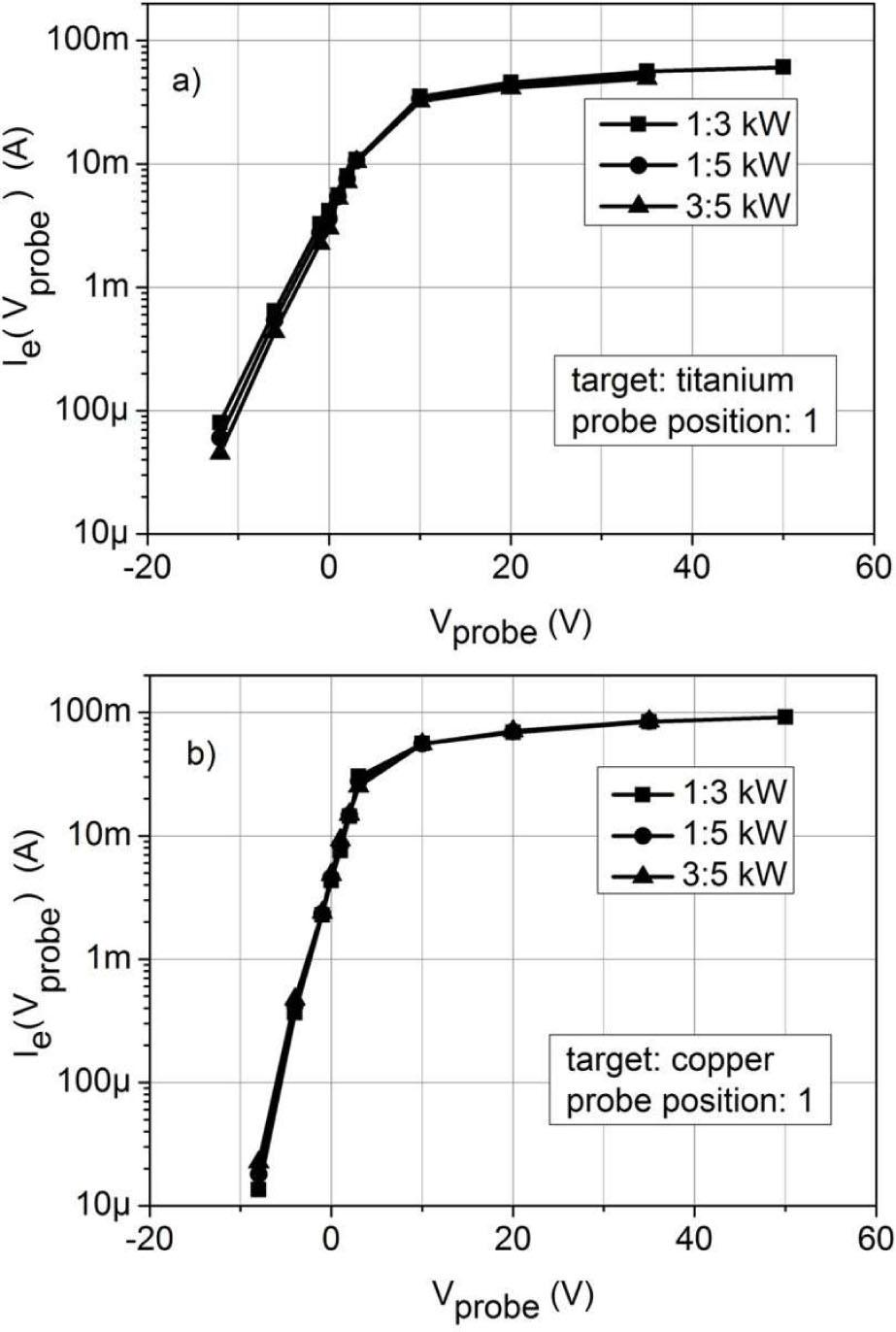The curves of probe electron current for (a) copper and (b) titanium sputtering processes at position 1, calculated with different value pairs of discharge power (PE1:PE2).
Calculations of plasma parameters

If EEDF may be assumed to be Maxwellian then the electron current collected by the probe s a function of probe voltage Vprobe may be expressed using simple and well-known equations. For the case of probe potential being lower than plasma potential Vprobe ⩽ Vp, the electron current collected by the probe is given by [18, 19, 41]:

$Ie(Vprobe)=Iesexp[e(Vprobe−Vp)kTe],Vprobe⩽Vp$$${I_e}({V_{probe}}) = {I_{es}}\exp \left[ {\frac{{e\left( {{V_{probe}} - {V_p}} \right)}}{{k{T_e}}}} \right],{V_{probe}} \leqslant {V_p}$$

For the case of probe potential being higher than plasma potential Vprobe > Vp, the saturation of electron current including the effect of sheath expansion, the electron current collected by the probe follows the equation [19, 31]:

$Ie(Vprobe)=Ies1+e(Vprobe−Vp)kTe,Vprobe>Vp$$${I_e}({V_{probe}}) = {I_{es}}\sqrt {1 + \frac{{e\left( {{V_{probe}} - {V_p}} \right)}}{{k{T_e}}}},{V_{probe}} > {V_p}$$

The electron saturation current Ies is expressed as Ies = 1/4(enevethAprobe),[18-20] where ne is the electron density, Te is the electron temperature, Vp is the plasma potential, Aprobe is the probe area and $veth≡8kTe/πme$${v_{{\rm{eth}}}} \equiv \sqrt {8k{T_e}/\pi {m_e}}$ is the electron thermal speed.

Basing on equation 6 and equation 7, the electron temperature, electron density and plasma potential may be calculated using semi-logarithmic plot of electron current versus probe voltage. Fitting the straight line through the retardation region one can find the electron temperature Te from equation 6. Using the same plot, the plasma potential Vp and electron saturation current Ies can be obtained from the intersection of the previous line with the straight line through electron saturation region: the intersection point coordinates are (Vp, Ies). It is convenient to calculate the electron density ne from equation 7 with the probe potential being equal to the plasma potential Vp.

Considering given cathode material and probe position, the discharge power increase causes the electron current curves to be shifted along the current axis (Fig. 4 and Fig. 5). The slope of the curves in the region of electron retardation seems to be unaffected by the discharge power level. Using the data from Fig. 4a (titanium sputtering, probe position No.1) one can obtain with equation 6 the electron temperature of about 2.7 eV for each of the three presented curves. Similarly, using the data from Fig. 4b (copper sputtering, probe position No.1) one can obtain the electron temperature of about 1.7 eV for each of the three presented curves. Finally, calculating electron temperatures using the data from Fig. 8 one can obtain values of 2.8 and 1.6 eV for titanium and copper sputtering (position No.1), respectively. This convergence of results confirms that the measured values of probe current are dependent on discharge power level (proportional to the ratio of group duration time to group repetition time) with a constant coefficient. The exact influence of this coefficient on the probe electron current is given by equation 4 and equation 5. Using equation 1, which is a simplified form of equation 4 and equation 5, and choosing two points on the linear part of electron current curve plotted semi-logarithmically with respect to the probe voltage (retardation of electrons) such that Vprobe2 >Vprobe1 from equation 6 one can easily obtain:

$Te−ekVprobe2−Vprobe1lnIe(Vprobe2,PE)Ie(Vprobe2,PE)=ekVprobe2−Vprobe1lnIe(Vprobe2)Ie(Vprobe2)$$${T_e} - \frac{e}{k}\frac{{{V_{probe2}} - {V_{probe1}}}}{{\ln \frac{{{I_e}\left( {{V_{probe2}},{P_E}} \right)}}{{{I_e}\left( {{V_{probe2}},{P_E}} \right)}}}} = \frac{e}{k}\frac{{{V_{probe2}} - {V_{probe1}}}}{{\ln \frac{{{I_e}\left( {{V_{probe2}}} \right)}}{{{I_e}\left( {{V_{probe2}}} \right)}}}}$$

Fortunately, the electron temperature is not perturbed by the magnetron current waveform influence, as the tgroup(PE)/Tgating ratio occurring in equation 1 cancels during calculations. This result is in quite good agreement with the observations published in  where electron temperature was found to be only slightly dependent on the discharge power, varying from 0.85 to 1.25 eV with the discharge power change from 0.5 to 4.5 kW, respectively. Summarizing, with respect to magnetron discharge powered by the presented medium frequency power supply, the electron temperature calculations give the reliable results, independent of discharge power level at which the probe I-V characteristics was measured.

The case is different when it comes to plasma density calculations. Unfortunately, taking equation 6 and equation 7 into account, and using the data from Fig. 4 and Fig. 5, the calculated electron density (plasma density) will be different at different discharge power levels, since equation 6 and equation 7 result with the plasma density to be equal to:

$ne=IeseAprobe2πmekTe$$${n_e} = \frac{{{I_{es}}}}{{e{A_{probe}}}}\sqrt {\frac{{2\pi {m_e}}}{{k{T_e}}}}$$

The electron saturation current Ies, being ordinate of the above mentioned intersection point (Vp, Ies), is influenced by the discharge power (influenced by the tgroup(PE)/Tgating ratio). Regarding to the diagnostics of titanium sputtering processes at position No. 1, using the data from Fig. 4a one can obtain the plasma density of about 0.5, 1.3, 1.9 × 1017 m–3 at discharge power of 1, 3, 5 kW, respectively, whereas the right plasma density (for the plasma present during the train of sputtering pulses, PE max), calculated using the data from Fig. 8a, is about 2.4 × 1017 m–3 Regarding to the diagnostics of copper sputtering processes at position No. 1, the data from Fig. 4b will result with the plasma density of about 0.7, 1.9, 3.3 × 1017 m–3 at discharge power of 1, 3, 5 kW, respectively, whereas the plasma density, calculated using data from Fig. 8b, is about 5.1 × 1017 m–3 At position No. 2 the plasma density of titanium sputtering processes was calculated to be 0.4, 0.8, 1.3, 1.6 × 1017 m–3 at discharge power of 1, 3, 5, 7.5 (PE_max) kW, respectively. With respect to copper sputtering processes, the plasma density at position No. 2 was calculated to be 0.5, 1.3, 2.3, 3.4 × 1017 m–3 at discharge power of 1, 3, 5, 9 (PE_max) kW, respectively. The plasma density calculated using data of probe I-V characteristics measured at discharge power of 1 kW, for both the titanium and copper sputtering processes, is about five times underestimated in comparison to the actual density obtained from probe I-V characteristics scaled up to the condition of magnetron powering by continuous train of pulses (Fig. 8). As the discharge start-up and decay phases have a different time constants for the titanium and copper sputtering processes, then the ratios of plasma density calculated at different discharge power levels differ.

Summary

The simplicity and cost of single Langmuir probe measurement equipment (especially systems for time-averaged measurements), makes this technique very attractive for basic diagnostics of magnetron plasma. Unfortunately, the measured data are often affected by the experimental conditions, effects of magnetic field presence, geometrical characteristics of probes and discharge power. The possibility of probe tip heating during measurements of electron saturation current causes the Langmuir probe measurements to be conducted at low discharge power. Unfortunately, with respect to pulsed magnetron powering using power supply with pulse density modulation, the Langmuir probe I-V characteristics are affected by the discharge power level, i.e. affected by the ratio of group duration time to group repetition time. In this paper, the impact of medium frequency pulsed magnetron discharge power on the average (direct current) I-V characteristics of single Langmuir probe and resulting plasma parameters was presented. Basing on measured DC probe I-V characteristics and time-resolved analysis of probe current, a method of results scaling up was elaborated. If a magnetron power supply uses the pulse density modulation, like the presented DPS power supply, then it is possible to calculate the reliable values of plasma parameters (not influenced by the discharge power level), using the presented approach.

Bräuer G., Szyszka B., Vergöhl M., Bandorf R., Vacuum, 84 (2010), 1354.BräuerGSzyszkaBVergöhlMBandorfRVacuum842010135410.1016/j.vacuum.2009.12.014Search in Google Scholar

Helmersson U., Lattemann M., Bohlmark J., Ehiasarian A.P., Gudmundsson J.T., Thin Solid Films, 513 (2006), 1.HelmerssonULattemannM Bohlmark JEhiasarianA.PGudmundssonJ.TThin Solid Films5132006110.1016/j.tsf.2006.03.033Search in Google Scholar

Musil J., Baroch P., Vlček J., Nam K.H., Han J.G., Thin Solid Films, 475 (2005), 208.MusilJBarochPVlčekJNamK.HHanJ.GThin Solid Films475200520810.1016/j.tsf.2004.07.041Search in Google Scholar

Kelly P.J., Arnell R.D., Vacuum, 56 (2000), 159.KellyP.JArnellR.DVacuum56200015910.1016/S0042-207X(99)00189-XSearch in Google Scholar

Musil J., Baroch P., Vacuum, 87 (2013), 96.MusilJBarochPVacuum8720139610.1016/j.vacuum.2012.02.023Search in Google Scholar

Baghriche O., Ehiasarian A.P., Kusiak-Nejman E., Pulgarin C., Sanjines R., Morawski A.W., Kiwi J., J.Photoch.Photobiol.A, 227 (2012), 11.BaghricheOEhiasarianA.PKusiak-NejmanEPulgarinCSanjinesRMorawskiA.WKiwiJJ.Photoch.Photobiol.A22720121110.1016/j.jphotochem.2011.10.017Search in Google Scholar

Anders A., Surf.Coat.Technol., 205 (2011), S1.AndersASurf.Coat.Technol2052011S110.1016/j.surfcoat.2011.03.081Search in Google Scholar

Lin J., Sproul W.D., Moorej.J., Wu Z., Lee S., Chistyakov R., Abraham B., Struct.Funct.Biol.ThinFilms, 63 (2011), 48.LinJSproulW.DMoorejJWuZLeeSChistyakovRAbrahamBStruct.Funct.Biol.ThinFilms6320114810.1007/s11837-011-0092-4Search in Google Scholar

Gudmundsson J.T., Vacuum, 84 (2010), 1360.GudmundssonJ.TVacuum842010136010.1016/j.vacuum.2009.12.022Search in Google Scholar

Anders A., Surf.Coat.Technol., 204 (2010), 2864.AndersASurf.Coat.Technol2042010286410.1016/j.surfcoat.2010.01.047Search in Google Scholar

Kelly P.J., West G., Kok Y.N., Bradley J.W., Swindells I., Clarke G.C.B., Surf.Coat.Technol., 202 (2007), 952.KellyP.JWestGKokY.NBradleyJ.WSwindellsIClarkeG.C.BSurf.Coat.Technol202200795210.1016/j.surfcoat.2007.04.130Search in Google Scholar

Anders A., Surf.Coat.Technol., 200 (2005), 1893.AndersASurf.Coat.Technol2002005189310.1016/j.surfcoat.2005.08.018Search in Google Scholar

Musil J., Kadlec S., Münz W.D., J.Vac.Sci.Tech-nol.A, 9 (1991), 1171.MusilJKadlecSMünzW.DJ.Vac.Sci.Tech-nol.A91991117110.1116/1.577597Search in Google Scholar

Rogozin A., Vinnichenko M., Shevchenko N., Kolitsch A., Müller W., Thin Solid Films, 496 Uller(2006), 197.RogozinAVinnichenkoMShevchenkoNKolitschAMüllerWThin Solid Films Uller496200619710.1016/j.tsf.2005.08.273Search in Google Scholar

Britun N., Han J.G., Thin Solid Films, 516 (2008), 6542.BritunNHanJ.GThin Solid Films5162008654210.1016/j.tsf.2007.11.027Search in Google Scholar

Britun N., Gaillard M., Oh S.G., Han J.G., J.Phys.D.Appl.Phys., 40 (2007), 5098.BritunNGaillardMOh S.GHanJ.GJ.Phys.D.Appl.Phys402007509810.1088/0022-3727/40/17/015Search in Google Scholar

Belkind A., Zhu W., Lopez J., Becker K., Plasma Sources Sci.T., 15 (2006), S17.BelkindAZhuWLopezJBeckerKPlasma Sources Sci.T152006S1710.1088/0963-0252/15/2/S03Search in Google Scholar

Bradley J.W., B äcker H., Kelly P.J., Arnell R.D., Surf.Coat.Technol., 135 (2001), 221.BradleyJ.WBäckerHKellyP.JArnellR.DSurf.Coat.Technol135200122110.1016/S0257-8972(00)00990-7Search in Google Scholar

Langmuir I., Mott-Smith H.M., Phys.Rev., 28 (1926), 727.LangmuirIMott-SmithH.MPhys.Rev28192672710.1103/PhysRev.28.727Search in Google Scholar

Merlino R.L., Am. J. Phys. 75 (2007), 1078.MerlinoR.LPhysAm. J752007107810.1119/1.2772282Search in Google Scholar

Chen F.F., Phys.Plasmas, 14 (2007), 094703.ChenF.FPhys.Plasmas14200709470310.1063/1.2772625Search in Google Scholar

Chen F.F., Phys.Plasmas, 8 (2001), 3029.ChenF.FPhys.Plasmas82001302910.1063/1.1368874Search in Google Scholar

Chen F.F., Evans J.D., Arnush D., Phys.Plasmas, 9 (2002), 1449.ChenF.FEvansJ.DArnushDPhys.Plasmas92002144910.1063/1.1462630Search in Google Scholar

Tichy M., Kudrna P., Behnke J.F., Csambal C., Klagge S., J.Phys., 7 (1997), 397.TichyMKudrnaPBehnkeJ.FCsambalCKlaggeSJ.Phys71997397Search in Google Scholar

Window B., Savvides N., J.Vac.Sci.Technol.A,4 (1986), 196.WindowBSavvidesNJ.Vac.Sci.Technol.A4198619610.1116/1.573470Search in Google Scholar

Samuell C.M., Blackwell B.D., Howard J., Corr C.S., Phys.Plasmas, 20 (2013), 034502.SamuellC.MBlackwellB.DHowardJCorrC.SPhys.Plasmas20201303450210.1063/1.4794841Search in Google Scholar

Johnson J.D., Holmes A.J.T., Rev.Sci.Instrum., 61 (1990), 2628.JohnsonJ.DHolmesA.J.TRev.Sci.Instrum611990262810.1063/1.1141849Search in Google Scholar

Spolaore M., Antoni V., Bagatin M., Buffa A., Cavazzana R., Desideri D., Martines E., Pomaro N., Serianni G., Tramontin L., Surf.Coat.Technol., 116 (1999), 1083.SpolaoreMAntoniVBagatinMBuffaACavazzanaRDesideriDMartinesEPomaroNSerianniGTramontinLSurf.Coat.Technol1161999108310.1016/S0257-8972(99)00122-XSearch in Google Scholar

Posadowski W.M., Thin Solid Films, 392 (2001), 201.PosadowskiW.MThin Solid Films392200120110.1016/S0040-6090(01)01028-8Search in Google Scholar

Brudnik A., Czapla A., Posadowski W., Vacuum, 82 (2008), 1124.BrudnikACzaplaAPosadowskiWVacuum822008112410.1016/j.vacuum.2008.01.029Search in Google Scholar

Straňäk V., HubičKa Z., AdáMek P., BlažEk J., TichÞ M.,ŠPatenka P., Hippler R., Wrehdes.,Surf.Coat.Technol.,201(2006),2512.StraňäkK VHubičKaZAdáMekPBlažEkJTichÞMŠPatenkaPHipplerRWrehdeSSurf.Coat.Technol2012006251210.1016/j.surfcoat.2006.04.013Search in Google Scholar

Liebig B., Braithwaite N., Kelly P.J., Chistyakov R., Abraham B., Bradley J.W., Surf.Coat.Technol., 205 (2011), S312.LiebigBBraithwaiteNKellyP.JChistyakovRAbrahamBBradleyJ.WSurf.Coat.Technol2052011S31210.1016/j.surfcoat.2011.01.017Search in Google Scholar

Gudmundsson J.T., Alami J., Helmersson U., Appl.Phys.Lett., 78 (2001), 3427.GudmundssonJ.TAlamiJHelmersson UAppl.Phys.Lett782001342710.1063/1.1376150Search in Google Scholar

Wiatrowski A., Vacuum, 82 (2008), 1111.WiatrowskiAVacuum822008111110.1016/j.vacuum.2008.01.027Search in Google Scholar

Gencoa Ltd.: Www.Gencoa.Com/Balance_And_ Unbalance/ (2015).Gencoa Ltd.: Www.Gencoa.Com/Balance_And_ Unbalance2015Search in Google Scholar

Dora J., Polish Patent No. 313150, 1996.DoraJPolish Patent No. 3131501996Search in Google Scholar

Depla D., Buyle G., Haemers J., De Gryse R., Surf.Coat.Technol., 200 (2006), 4329.DeplaDBuyleGHaemersJDe GryseRSurf.Coat.Technol2002006432910.1016/j.surfcoat.2005.02.166Search in Google Scholar

Bradley J.W., B äcker H., Surf.Coat.Technol., 200 (2005), 616.BradleyJ.WB ¨ AckerHSurf.Coat.Technol200200561610.1016/j.surfcoat.2005.01.074Search in Google Scholar

Bradley J.W., B äcker H., Kelly P.J., Arnell R.D., Surf.Coat.Technol., 142 (2001), 337.BradleyJ.WBäckerHKellyP.JArnellR.DSurf.Coat.Technol142200133710.1016/S0257-8972(01)01084-2Search in Google Scholar

Drache S., Stranak V., Herrendorf A., Cada M., Hubicka Z., Tichy M., Hippler R., Vacuum, 90 (2013), 176.DracheSStranakVHerrendorfACadaMHubickaZTichyMHipplerRVacuum90201317610.1016/j.vacuum.2012.02.047Search in Google Scholar

Poolcharuansin P., Bradley J.W., Plasma Sources Sci.T, 19 (2010), 025010.PoolcharuansinPBradleyJ.WPlasma Sources Sci.T19201002501010.1088/0963-0252/19/2/025010Search in Google Scholar

Passoth E., Kudrna P., Csambal C., Behnke J.F., Tich Þ; M., Helbig V., J.Phys.D:Appl.Phys, 30 (1997), 1763.PassothEKudrnaPCsambalCBehnkeJ.FTichÞMHelbig VJ.Phys.D:Appl.Phys301997176310.1088/0022-3727/30/12/013Search in Google Scholar

Rossnagel S.M., J.Vac.Sci.Technol.A, 6 (1988), 1821.RossnagelS.MJ.Vac.Sci.Technol.A61988182110.1116/1.575262Search in Google Scholar

Helmersson U., Lattemann M., Bohlmark J., Ehiasarian A.P., Gudmundsson J.T., Thin Solid Film, 513 (2006), 1.HelmerssonULattemannMBohlmarkJEhiasarianA.PGudmundssonJ.TThin Solid Film5132006110.1016/j.tsf.2006.03.033Search in Google Scholar

Chen F.F., Lecture Notes on Langmuir Probe Diagnostics, IEEE-ICOPS meeting, Mini-Course on Plasma Di-agnostics, Jeju, Korea, June 2003, http://www.seas. ucla.edu/~ffchen/publications.htm (2015).ChenF.FLecture Notes on Langmuir Probe DiagnosticsIEEE-ICOPS meeting, Mini-Course on Plasma Di-agnostics, Jeju, Korea, June 2003http://www.seas. ucla.edu/~ffchen/publications.htm2015Search in Google Scholar

• #### Growth, spectral, optical, dielectric and third order nonlinear studies on 2-amino 4-methylpyridinium salicylate single crystals

Recommended articles from Trend MD